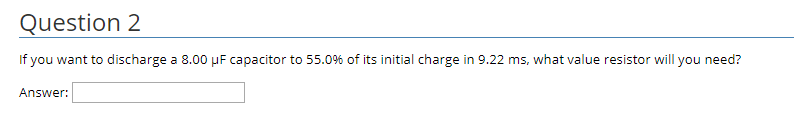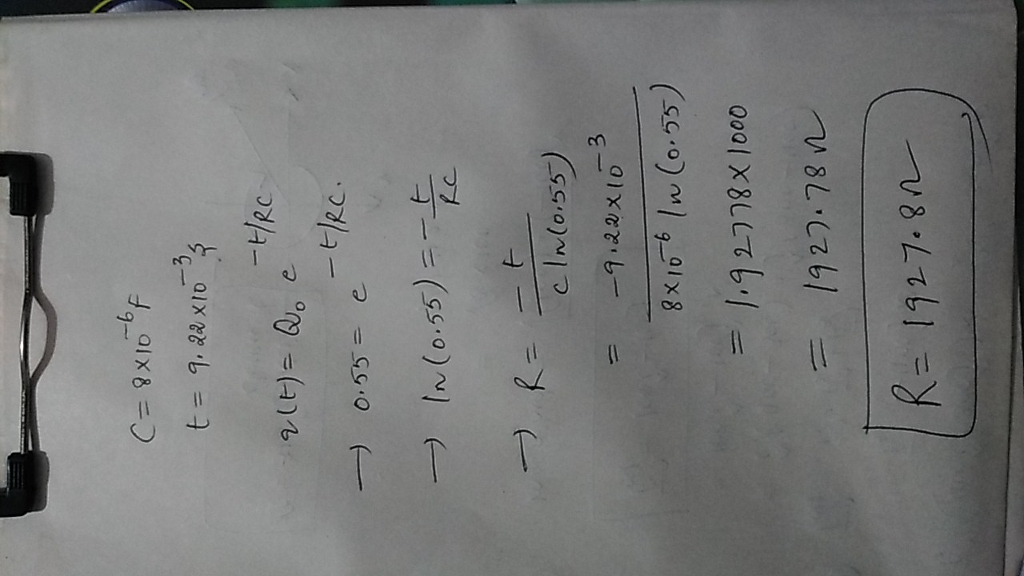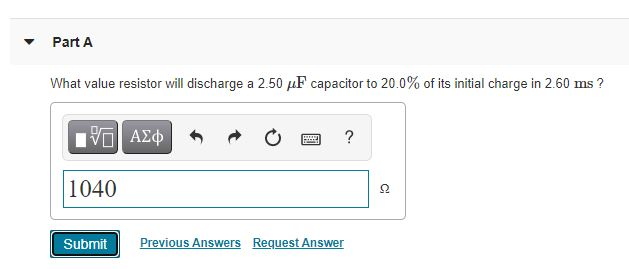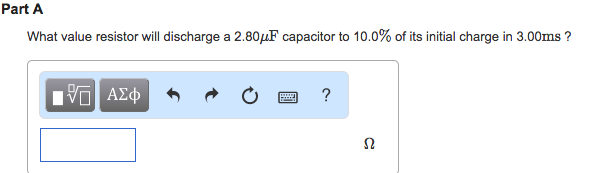# If you want to discharge a 8.00 μF capacitor to 55.0% of its initial charge in...

If you want to discharge a 8.00 μF capacitor to 55.0% of its initial charge in 9.22 ms, what value resistor will you need?Question 2 If you want to discharge a 8.00 μ F capacitor to 55.0% of its initial charge in 9.22 ms, what value resistor will you need? Answer:#### Earn Coin

Coins can be redeemed for fabulous gifts.

Similar Homework Help Questions
• ### What value resistor will discharge a 2.40 μF capacitor to 20.0% of its initial charge in...

What value resistor will discharge a 2.40 μF capacitor to 20.0% of its initial charge in 3.00 ms ?

• ### What value resistor will discharge a 2.1 μF capacitor to 20 % of its initial charge...

What value resistor will discharge a 2.1 μF capacitor to 20 % of its initial charge in 2.4 ms ?

• ### What value resistor will discharge a 3.0 μF capacitor to 10 % of its initial charge...

What value resistor will discharge a 3.0 μF capacitor to 10 % of its initial charge in 2.1 ms ?

• ### What value resistor will discharge a 2.1 μF capacitor to 20 % of its initial charge...

What value resistor will discharge a 2.1 μF capacitor to 20 % of its initial charge in 2.8 ms ?

• ### What value resistor will discharge a 2.60 μF capacitor to 20.0% of its initial charge in 2.50 ms?

What value resistor will discharge a 2.60 μF capacitor to 20.0% of its initial charge in 2.50 ms?

• ### What value resistor will discharge a 2.60 μF capacitor to 20.0 % of its initial charge in 2.50 ms

Part AWhat value resistor will discharge a 2.60 μF capacitor to 20.0 % of its initial charge in 2.50 ms?

• ### What value resistor will discharge a 2.6?F capacitor to 20% of its initial charge in 2.9ms...

What value resistor will discharge a 2.6?F capacitor to 20% of its initial charge in 2.9ms ? PLEASE ONLY SERIOUS INQUIRIES ONLY THIS IS MY GRADE AND I WANT TO LEARN HOW TO DO IT RIGHT STEP BY STEP Express your answer using two significant figures. R =   ?

• ### Part A What value resistor will discharge a 2.50 uF capacitor to 20.0% of its initial...Part A What value resistor will discharge a 2.50 uF capacitor to 20.0% of its initial charge in 2.60 ms? IVO AO ? 1040 2 Submit Previous Answers Request Answer

• ### What value resistor will discharge a 2.80μF capacitor to 10.0% of its initial charge in 3.00ms ? Part A What value r...What value resistor will discharge a 2.80μF capacitor to 10.0% of its initial charge in 3.00ms ?Part A What value resistor will discharge a 2.80 MuF capacitor to 10.0% of its initial charge in 3.00ms?

• ### What value resistor will discharge a 3.00microfarads capacitor to 20.0% of its initial charge in...

What value resistor will discharge a 3.00microfarads capacitor to 20.0% of its initial charge in 2.10ms ?